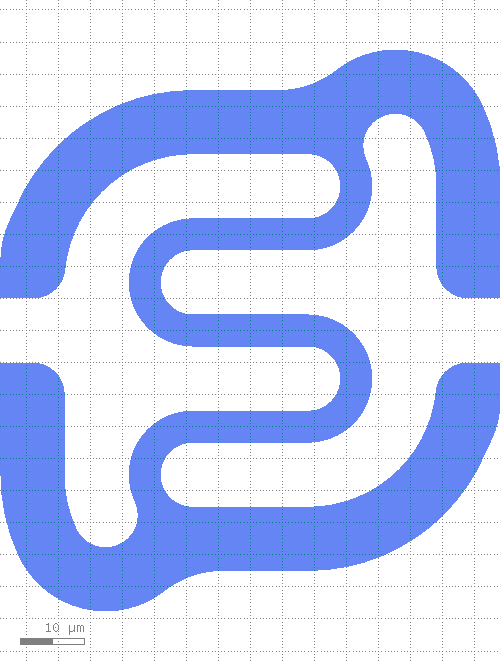# kqcircuits.elements.smooth_capacitor¶

class kqcircuits.elements.smooth_capacitor.SmoothCapacitor[source]

The PCell declaration for a smooth finger capacitor.

SmoothCapacitor is a finger capacitor, which has continuous geometry changes through the capacitance range. This leads to continuous capacitance function, which enables using capacitor inside numerical optimization methods.

Capacitance range is achieved by changing single parameter called finger_control.

can_create_from_shape_impl()[source]

default implementation

build()[source]

Child classes re-define this method to build the PCell.

PCell parameters:

• finger_control (Double) - Parameter for capacitor growth (related to number of fingers per side), default=`2.1`

• ground_gap (Double) - Gap between ground and finger, default=`10`, unit=`μm`

• a2 (Double) - Non-physical value ‘-1’ means that the default size ‘a’ is used., default=`-1`, unit=`μm`

• b2 (Double) - Non-physical value ‘-1’ means that the default size ‘b’ is used., default=`-1`, unit=`μm`

• fixed_length (Double) - Fixed length of element, 0 for auto-length, default=`0`, unit=`μm`

• finger_width (Double) - Width of a finger, default=`10`, unit=`μm`

• finger_gap (Double) - Gap between the fingers, default=`5`, unit=`μm`